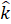Daily Practice Problems
Class 11 Physics
Work Energy and PowerQuestion 1.

Find the angle between force F = (2i+ 3j- 4k)unit and displacement d = (3i+ 5j+ 4k)unit. Also, find the projection of F and d.

Question 2.

A cyclist comes to a skidding stop in 12 m. During this process, the force on the cycle due to the road is 300N and is directly opposed to the motion.

1. How much work does the road do on the cycle?
2. How much work does the cycle do on the road?

Question 3.

Give units, symbol and dimensions for kinetic energy and potential energy.

Question 4.

A body constrained to move along the z-axis of a coordinate system is subject to a constant force F given by;

F = -2i+ 3j+ 2kN.

Where i, j, kare unit vectors along the x-, y- and z-axis of the system respectively. What is the work done by this force in moving the body a distance of 3 m along the z-axis?

Question 5.

A molecule in a gas container hits a horizontal wall with speed 300 m/s and angle 300 with the normal, and rebounds with the same speed. Is momentum conserved in the collision? Is the collision elastic or inelastic?

Question 6.

A pump on the ground floor of a building can pump up water to fill a tank of volume 27 m3 in 9 min. If the tank is 30 m above the ground, and the efficiency of the pump is 20%, how much electric power is consumed by the pump?

Question 7.

The bob of a pendulum is released from a horizontal position. If the length of the pendulum is 2.0 m. What is the speed with which the bob arrives at the lowermost point, given that it dissipated 10% of its initial energy against air resistance?

Question 8.

A trolley of mass 500 kg carrying a sandbag of 50 kg is moving uniformly with a speed of 30 km/h on a frictionless track. After a while, sand starts leaking out of a hole on the floor of the trolley at the rate of 0.04 kg/s. What is the speed of the trolley after the entire sandbag is empty?

Question 9.

A bolt of mass 0.5 kg falls from the ceiling of an elevator moving down with an uniform speed of 10 m/s. It hits the floor of the elevator (length of the elevator = 5 m) and does not rebound. What is the heat produced by the impact? Would your answer be different if the elevator were stationary?

Question 10.

A trolley of mass 300 kg moves with a uniform speed of 48 km/h on a frictionless track. A child of mass 30 kg runs on the trolley from one end to the other (15 m away) with a speed of 5 m/s relative to the trolley in a direction opposite to its motion, and jumps out of the trolley. What is the final speed of the trolley? How much has the trolley moved from the time the child begins to run?

Question 11.

A bullet of mass 0.015 kg and horizontal speed 55 m/s strikes a block of wood of mass 0.5 kg and instantly comes to rest with respect to the block. The block is suspended from the ceiling by means of thin wires. Calculate the height to which the block rises. Also, estimate the amount of heat produced in the block.

Question 12.

When will you say that a force is conservative?

Question 13.

State Work- Energy theorem.

Question 14.

A family uses 10 kW of power. Direct solar energy is incident on the horizontal surface at an average rate of 300 W per square meter. If 15% of this energy can be converted to useful electrical energy, how large an area is needed to supply 10 kW?

Question 15.

Give dimension of spring constant.

Question 16.

A body of mass 0.3 kg travels in a straight line with velocity, v = ay3/2, where a = 3 m/s2. What is the work done by the net force during its displacement from x = 0 to 3 m.

Question 17.

How can you write mechanical energy in terms of kinetic and potential energies?

Question 18.

A rain drop of radius 1 mm falls from a height of 400 m above the ground. It falls with decreasing (due to viscous resistance of the air) until at half its original height. It attains its maximum(terminal) speed, and moves with uniform speed thereafter. What is the work done by the resistive force in the entire journey if its speed on reaching the ground is 15 m/s.

Question 19.

What are the unit, symbol and dimension of work done?

Question 20.

A body constrained to move along the z-axis of a coordinate system is subject to a constant force F is given by:

F = 2i+ 3j+ 4kN

where i, jand kare unit vectors along x, y- and z-axis of the system respectively. What is the work done by this force in moving the body a distance of 5 m along the z-axis?

**********